[Next]: Dissipative Schrödinger-Poisson systems
 [Up]: Project descriptions
 [Previous]: Project descriptions
[Contents]   [Index]

## Simulation of the modulation response of strained multi-quantum-well lasers

Collaborator: U. Bandelow , H. Gajewski , A. Glitzky , R. Hünlich

Cooperation with: F. Fidorra, M. Krieg (MergeOptics GmbH, Berlin)

Supported by: MergeOptics GmbH

Description: In cooperation with the company MergeOptics GmbH the electrical, thermal, optical and dynamical behavior of strained multi-quantum-well (SMQW) lasers was simulated for improving their performance. The simulations were carried out using the device simulator WIAS-TeSCA based on a drift-diffusion model which is self-consistently coupled to a heat-flow equation (see ) and to equations for the optical field. The main goal was the optimization of the modulation response of such lasers. For this purpose details of the structure (e.g., number of quantum wells, thickness of waveguides) and the doping were varied.

Methods and results are demonstrated here for some fictive edge-emitting ridge waveguide (RW) laser. The most important part of its transverse cross section is shown in Figure 1, the simulated P-I characteristic in Figure 2. Besides RW lasers we investigated also buried heterostructure lasers in more detail.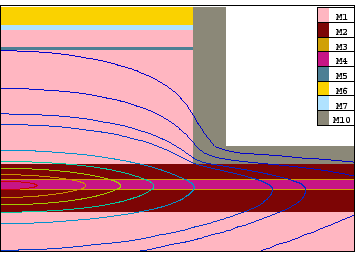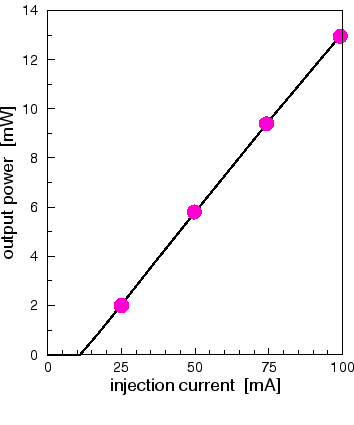We describe two methods for the calculation of the modulation response. The first one is the AC analysis (small signal analysis in the frequency domain). Here WIAS-TeSCA computes complex amplitudes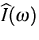,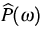corresponding to a small harmonic modulation of the bias voltage with angular frequency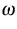and amplitude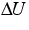(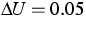mV). The complex response function is then given by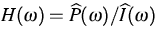, or in a normalized form by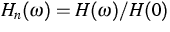. The squared modulus of Hn is seen in Figure 4. An excellent fit of Hn can be obtained according to the formula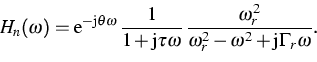(1)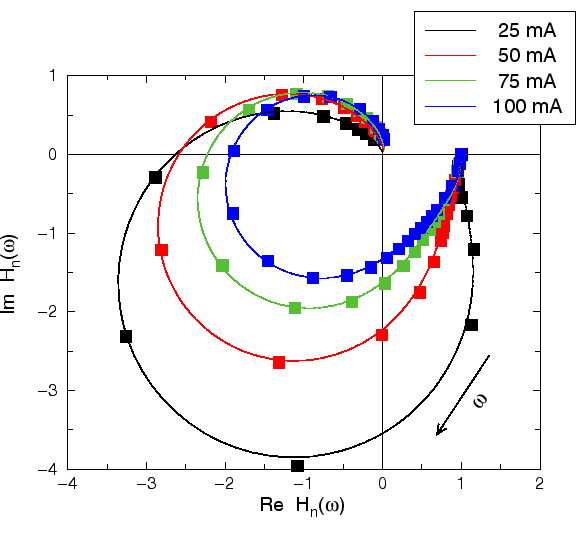The last factor in (1) is well known from rate equation models (see ). The second factor is a low-pass filter while the first one describes some time delay. Both terms are due to parasitic effects, especially to the drift-diffusion transport through the device (see , too). The result of the fit is demonstrated in Figure 3. The extracted parameters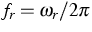,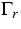,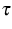and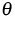are presented in a more suitable form in Figure 5. The frequency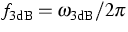is also shown there where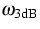solves the equation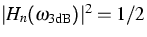.Let us note that a fit without using the parasitic terms gives insufficient results.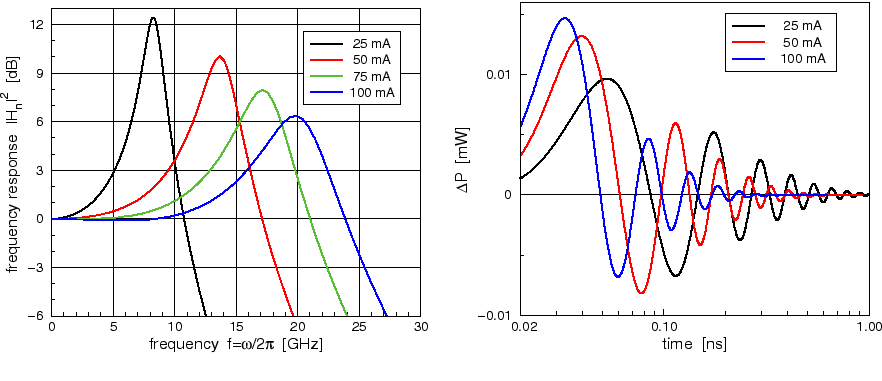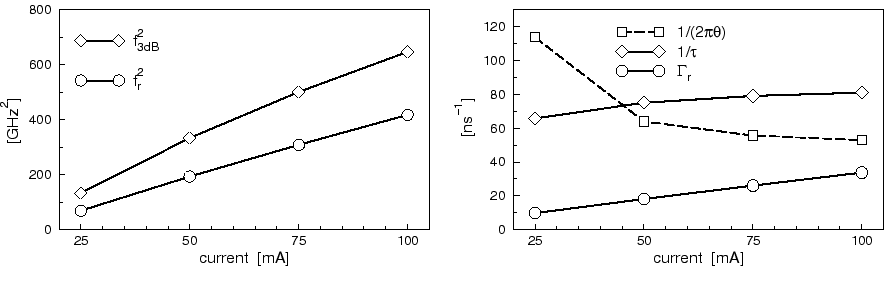The second method is a current-driven transient simulation where a short rectangular pulse is added to the injection current (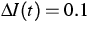mA,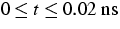). WIAS-TeSCA computes corresponding variations of the output power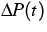which are seen in the right-hand side of Figure 4 for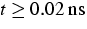(to enhance clearness the result for I=75 mA was omitted here). Corresponding to (1) the functions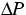were fitted according to the formula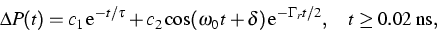(2)
where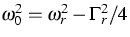, and c1, c2,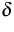depend on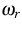,,,. The parameters extracted in this manner coincide well with the values found by the first method, and with (1) nearly the same frequency response functions as on the left hand side of Figure 4 are obtained. But let us note that this method requires very small time steps in order to get correct results.

References:

1. G. ALBINUS, H. GAJEWSKI, R. HÜNLICH, Thermodynamic design of energy models of semiconductor devices, Nonlinearity, 15 (2002), pp. 367-383.
2. S.L. CHUANG, Physics of Optoelectronic Devices, Wiley & Sons, New York, 1995.
3. M. KRIEG, Modellierung von Gewinnsättigung und Transporteffekten in Quantenfilmlasern mit Bilanzgleichungen, Shaker, Aachen, 2001.

 [Next]: Dissipative Schrödinger-Poisson systems
 [Up]: Project descriptions
 [Previous]: Project descriptions
[Contents]   [Index]

LaTeX typesetting by I. Bremer
5/16/2003